# eco331 practice finals solutions .pdf

### File information

Original filename: eco331-practice-finals-solutions.pdf

This PDF 1.4 document has been generated by TeX / pdfTeX-1.40.10, and has been sent on pdf-archive.com on 13/12/2010 at 19:32, from IP address 75.185.x.x. The current document download page has been viewed 976 times.
File size: 167 KB (5 pages).
Privacy: public file

eco331-practice-finals-solutions.pdf (PDF, 167 KB)

### Document preview

Northwestern University
Fall 2008

Marciano Siniscalchi
Econ 331-0

PRACTICE PROBLEMS SOLUTIONS

1. QUESTION 1 (medium). 15 points
We must argue by backward induction. Consider Ann first. Let α be her choice at time 0. If
p˜ = 0.4, then r˜1 : (1.15, 12 ; 0.9, 21 ) and r˜2 : (1.15, 0.4; 0.9, 0.6); thus, Ann will choose asset 1 [this is
by first-order stochastic dominance, or because expected utility is linear in the probabilities; some
argument must be provided!]. If instead p˜ = 0.54, then she will choose asset 2. Hence, given α,
Ann maximizes


1p
4 1p
W [(1 − α)1.02 + α1.15] +
W [(1 − α)1.02 + α0.9] +
5 2
2


p
p
1
+ 0.54 W [(1 − α)1.02 + α1.15] + 0.46 W [(1 − α)1.02 + α0.9] .
5
It is clear that W does not affect the decision, so we can cancel it. Collecting utilities, the objective
function becomes




p
p
41 1
41 1
+ 0.54
(1 − α)1.02 + α1.15 +
+ 0.46
(1 − α)1.02 + α0.9 =
52 5
52 5
p
p
=0.508 (1 − α)1.02 + α1.15 + 0.492 (1 − α)1.02 + α0.9.
Differentiating w.r.to α, setting the result to zero and rearranging yields
0.12
0.13
= 0.246 p
.
0.254 p
(1 − α)1.02 + α1.15
(1 − α)1.02 + α0.9
Further rearrangement yields
s
(1 − α)1.02 + α0.9
= 0.894003634
(1 − α)1.02 + α1.15

1.02 − 0.12α = 0.799242498[1.02 + 0.13α]

and finally
1.02(1 − 0.799242498)
= 0.914565689.
0.12 + 0.799242498 · 0.13
Now consider Bob’s problem. He will “reduce” probabilities and conclude that asset 2 has a
return of 1.10 with probability E[˜
p] = 45 · 0.4 + 15 · 0.54 = 0.428 &lt; 21 , and therefore he will decide to
invest in Asset 1 at time 1. At time 0, Bob’s problem is thus pretty standard: he maximizes
1p
1p
W [(1 − β)1.02 + β1.15] +
W [(1 − β)1.02 + β0.9].
2
2

Dividing by 12 W and differentiating yields
α=

1
0.13
1
0.12
p
− p
=0
2 (1 − β)1.02 + β1.15 2 (1 − β)1.02 + β0.9
1

2

PRACTICE PROBLEMS SOLUTIONS

Rearranging and squaring yields
(1 − β)1.02 + β0.9
= 0.852071006 ⇔ 1.02 − 0.12β = 0.869112426 + 0.110769231 ∗ β
(1 − β)1.02 + β1.15
1.02 − 0.869112426
= 0.653846153.
⇔β=
0.12 + 0.110769231
2. QUESTION 2 (medium). 10 points for (a), 5 points for (b).
(a) It’s easy to do this by induction—in fact, “backward induction,” in a way, although there is
no decision to be made except at time 0. Conditional on X1 = x1 , . . . , Xn−1 = xn−1 , we must have
E[u(W0 +

n
X

Xi )|X1 = x1 , . . . , Xn−1 = xn−1 ] =

i=1

=E[u(W0 + x1 + . . . + xn−1 + Xn )|X1 = x1 , . . . , Xn−1 = xn−1 ] =
=E[u(W0 + x1 + . . . + xn−1 + Xn )] &lt; u(W0 + x1 + . . . + xn−1 ) :
the first equality holds because we are conditioning on the values of the first n − 1 repetitions,
the second equality follows from the fact that the Xi ’s are i.i.d., and the inequality follows from
the assumption that X1 and Xn have the same distribution and E[u(W + X1 )] &lt; u(W ) for all
W ∈ [W0 − nL, W0 + nG].
Now, by the law of total probability,
E[u(W0 + X1 + . . . + Xn )] =
=

X
xi ∈{G,L},i=1,...,n−1

&lt;

X

E[u(W0 +

n
X

Xi )|X1 = x1 , . . . , Xn−1 = xn−1 ] Pr[X1 = x1 , . . . , Xn−1 = xn−1 ] &lt;

i=1

u(W0 + x1 + . . . + xn−1 )] Pr[X1 = x1 , . . . , Xn−1 ] =

xi ∈{G,L},i=1,...,n−1

=E[u(W0 + X1 + . . . + Xn−1 )].
In other words, you’d rather take one fewer repetition of the bet. It is clear that repeating this
argument yields the result. Formally, suppose that you have shown that E[u(W0 +X1 +. . .+Xm )] &lt;
E[u(W0 + X1 + . . . + Xm−1 )] for some m ∈ 1, . . . , n [the above argument proves it for m = n]. If
m = 1, we are done [in this case, W0 +X1 +. . .+Xm−1 means W0 , according to standard conventions].
Otherwise, note that the above arguments applies verbatim with “m” in lieu of “n”: in particular,
the condition E[u(W +X1 )] &lt; u(W ) holds for any W ∈ [W0 −mL, W0 +mG] ⊂ [W0 −nL, W0 +nG],
because m ≤ n. Hence, we conclude that E[u(W0 +X1 +. . .+Xm−1 )] &lt; E[u(W0 +X1 +. . .+Xm−2 )]:
if the above inequality holds for m, it also holds for m−1. This completes the proof of the inductive
step.

5
200 + 21 100
(b) We have E[u(W0 + X1 )] = 12 200 + 12
 1= 191.666 . . . &lt; 200 = u(W0 ), but
1
5
1
5
E[u(W0 + X1 + X2 )] = 4 200 + 12 400 + 2 200 + 12 100 + 4 · 0 = 212.5 &gt; 200 = u(W0 ).
The reason this is consistent with (a) is that, if wealth is at least W0 + 200 = 400, which includes
a whole interval within the range [W0 − 2 · 100, W0 + 2 · 200] = [0, 600], then the individual will
evaluate the single instance of the bet using linear preferences, and in this case she will obviously
accept it. So, the conditions in (a) are violated.

PRACTICE PROBLEMS SOLUTIONS

3

3. QUESTION 3 (easy). 10 points.
Suppose that Varα [X] ≤ Varα [Y ] for all α ∈ [0, 1]. We show that Y FOSD X. Pick x ∈ R: then
by assumption VarFX (x) [X] ≤ VarFX (x) [Y ], i.e. x = FX−1 (FX (x)) ≤ FY−1 (FX (x)), and therefore
FY (x) ≤ FX (x), i.e. 1 − FY (x) ≥ 1 − FX (x); it follows that, for all W , W − X FOSD W − Y , and
the claim follows from the characterization of FOSD we proved in class.
In the other direction, the utility characterization implies that W − X FOSD W − Y , so Y
FOSD X. Choose α: then FX (Varα [X]) ≥ FY (Varα [X]), i.e. by definition α ≥ FY (FX−1 (α)), i.e.
VaRα [Y ] = FY−1 (α) ≥ FX−1 (α) = VaRα [X], as required.
4. QUESTION 4
(a) We must have (multiplying by −1)
1
1
e−λW = e−λ(W −p+50) + e−λ(W −p) .
2
2
λW
Multiply both sides by 2e
to get
2 = eλp−λ50 + eλp
Therefore
2
eλp = −λ50
e
+1
2
i.e. plugging in, e0.001p = e−0.05
=
1.02499479,
so
0.001p = 0.0246875297 and therefore p ≈
+1
24.6875.
(b) Now we need
1
1
W 1−γ = (W − p + 50)1−γ + (W − p)1−γ .
2
2
Plugging in γ = 2 and p = 15, we get
1
1
W −1 = (W + 35)−1 + (W − 15)−1 .
2
2
Multiplying by 2W yields
2=

W
W 2 − 15W + W 2 + 35W
W
+
=
W + 35 W − 15
W 2 + 20W − 525

hence
2W 2 + 40W − 1050 = 2W 2 + 20W

W = 52.5.

5. QUESTION 5
(a) Denote by πt the probability that the store assigns to Scenario 1 at the beginning of time
t, as a function of its observations to date. Thus, π1 = 0.6 and π2 is a random variable whose
realization depends upon the store’s choice of product and whether or not there was a sale.
We use backward induction. At time 2, the store must choose myopically: it will offer A if π2 ≥ 12
and B otherwise. Therefore, if π2 ≥ 21 , the store’s expected payoff will be
πt [0.75 · 1 + 0.25 · 0] + (1 − πt )[0.75 · 0 + 0.25 · 1] = πt 0.75 + (1 − πt )0.25 = 0.25 + 0.5πt ;
if instead π2 &lt; 12 , then the expected payoff is
(1 − πt )(0.75 · 1 + 0.25 · 0) + πt (0.75 · 0 + 0.25 · 1) = (1 − πt )0.75 + πt 0.25 = 0.75 − 0.5πt .

4

PRACTICE PROBLEMS SOLUTIONS

Now consider time 1. If the store chooses A and a sale occurs, then the store will have a payoff
of 1 at time 1, and update her beliefs about the likelihood of Scenario 1 using Bayes’ rule:
0.75 · 0.6
π2 =
= .81818181818181818181,
0.75 · 0.6 + 0.25 · 0.4
so the store will continue to choose A at time 2. If instead a sale does not occur, then there is no
payoff at time 0, and furthermore
0.25 · 0.6
π2 =
= .33333333333333333333
0.25 · 0.6 + 0.75 · 0.4
so that the store will choose B at time 2. Finally, the ex-ante probability of a sale at time 1 if the
store chooses A is
0.75 · 0.6 + 0.25 · 0.4 = .55
and therefore the total expected payoff from choosing A at time 1 is (plugging in the formulas for
payoff at time 2)
0.55 · (1 + 0.25 + 0.5 · .8181818181818181) + 0.45 · (0 + 0.75 − 0.5 · .3333333333333333) = 1.175.
If instead the store chooses B, and a sale occurs, then
0.25 · 0.6
= .33333333333333333333
π2 =
0.25 · 0.6 + 0.75 · 0.4
so the store will continue with B; and if a sale does not occur at time 1, then
0.75 · 0.6
π2 =
= .8181818181818181
0.75 · 0.6 + 0.25 · 0.4
so A will be optimal in the continuation (note the symmetry). The probability of a sale is
0.25 · 0.6 + 0.75 · 0.4 = .45
Hence, the total expected payoff from B at time 1 is
.45 · (1 + 0.75 − 0.5 · 0.3333333333333333) + 0.55 · (0 + 0.25 + 0.5 · 0.8181818181818181) = 1.075.
It is therefore optimal to choose A at time 1.
(b) Yes, it turns out that the myopic policy is optimal in this case.
6. QUESTION 6
(a) Let X : (150, 0.4; 80, 0.6). Without any newsletter, the investor compares
−0.4e−λ(W +(150−100)50) − 0.6e−λ(W +(80−100)50) .
with −e−λW . Dividing by e−λW and plugging in λ = 0.001, she compares
−0.4e−0.001·2500 − 0.6e0.001·1000 = −0.4e−2.5 − 0.6e1 = −1.663803
with −1. Thus, she should not buy the shares.
(b) Let’s consider BadNews first. We need to compute the probability of H in case of a bad
report:
Pr[X = H|B = L] =

0.4 · 0.4
Pr[B = L|X = H] Pr[X = H]
=
= 0.2285714.
Pr[B = L|X = H] Pr[X = H] + Pr[B = L|X = L] Pr[X = L]
0.4 · 0.4 + 0.9 · 0.6

PRACTICE PROBLEMS SOLUTIONS

5

Hence, in case of a bad report, we are comparing
−0.2285714e−2.4 − (1 − 0.2285714)e1.1 = −2.33823512
with −e−λ·(−100) = −e0.1 = −1.10517092, because at this stage we have already paid for the report;
thus, we would not buy; in case of a good report,
0.6 · 0.4
Pr[B = H|X = H] Pr[X = H]
Pr[X = H|B = H] =
=
= 0.8,
Pr[B = H|X = H] Pr[X = H] + Pr[B = H|X = L] Pr[X = L]
0.6 · 0.4 + 0.1 · 0.6
and we compare −1.10517092 with
−0.8e−2.4 − 0.2e1.1 = −0.673407567
so in this case we do buy. In other words, if the investor gets the BadNews signal, she does not
buy in case of bad news (B = L), and does buy in case of good news (B = H). Now the ex-ante
probability of good news is
Pr[B = H] = Pr[B = H|X = H] Pr[X = H]+Pr[B = H|X = L] Pr[X = L] = 0.6·0.4+0.1·0.6 = 0.3,
so the ex-ante expected payoff in case the investor buys the BadNews signal is
0.3 · (−0.673407567) + 0.7 · (−1.10517092) = −0.975641914
which is better than not buying the signal and taking the optimal decision in (a), namely not
buying the shares (recall this nets utility −1).
Now let’s consider GoodNews. In case of a H signal,
0.9 · 0.4
= 0.6,
Pr[X = H|G = H] =
0.9 · 0.4 + 0.4 · 0.6
and we compare
−0.6e−2.45 − 0.4e1.05 = −1.1948366
with −e0.05 = −1.0512711: thus, with a H signal, the investor does not buy. A fortiori, she will
not buy in case of a L signal, as in that case
0.1 · 0.4
Pr[X = H|G = L] =
= 0.1
0.1 · 0.4 + 0.6 · 0.6
i.e. the probability of a good outcome is lower than if G = H. Hence, the investor will not buy no
matter what the realization of G, which means that G is worthless. So, she will not buy GoodNews.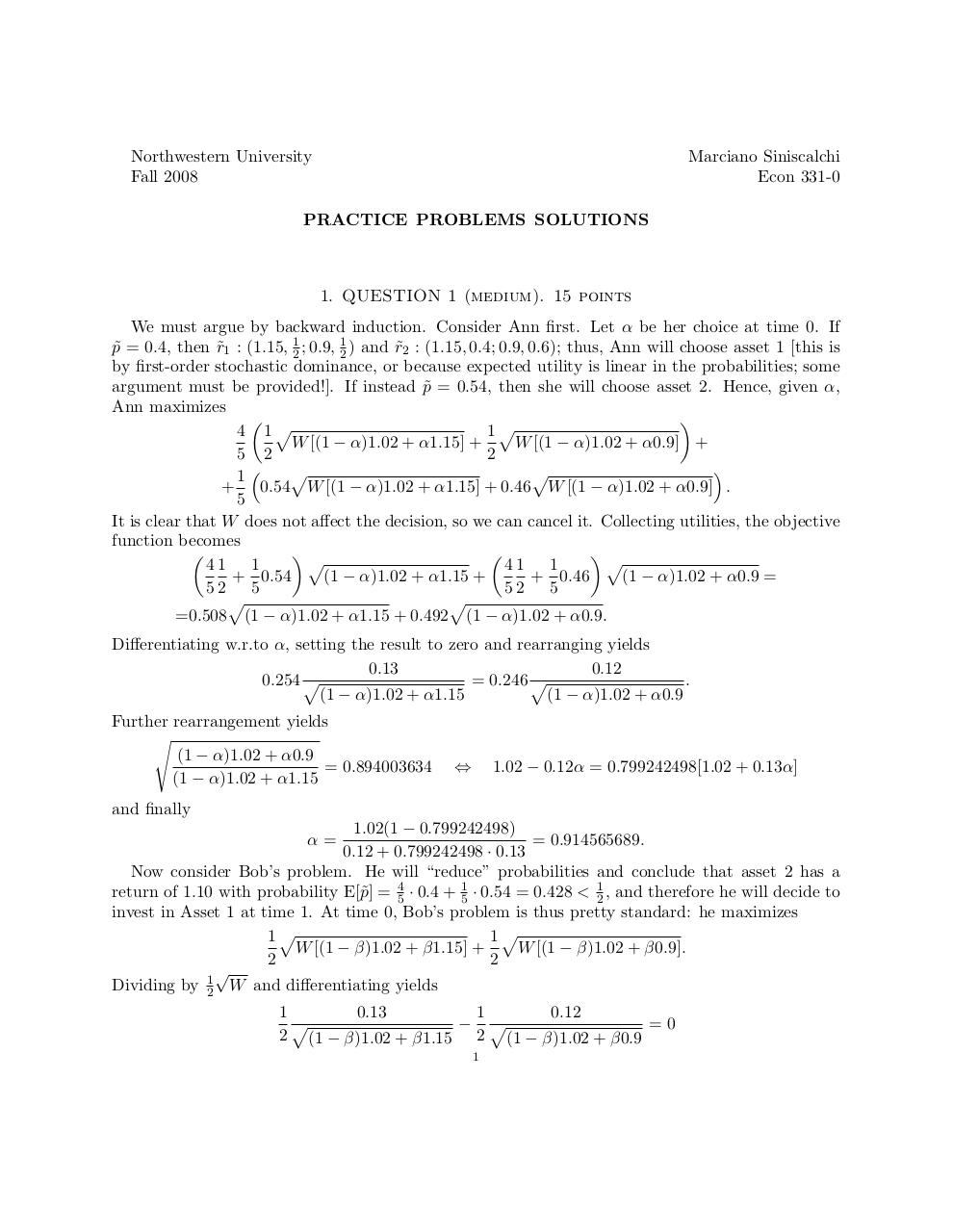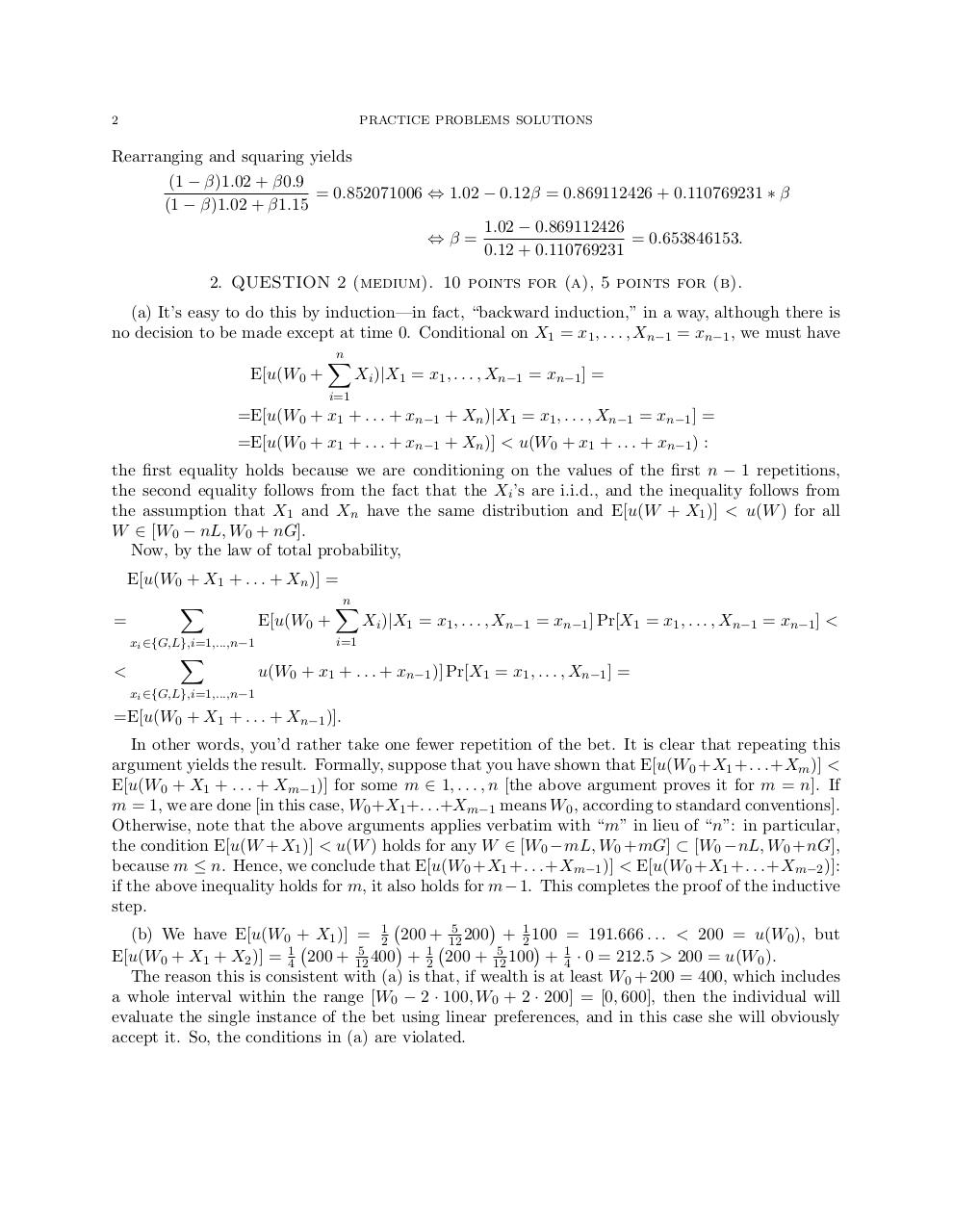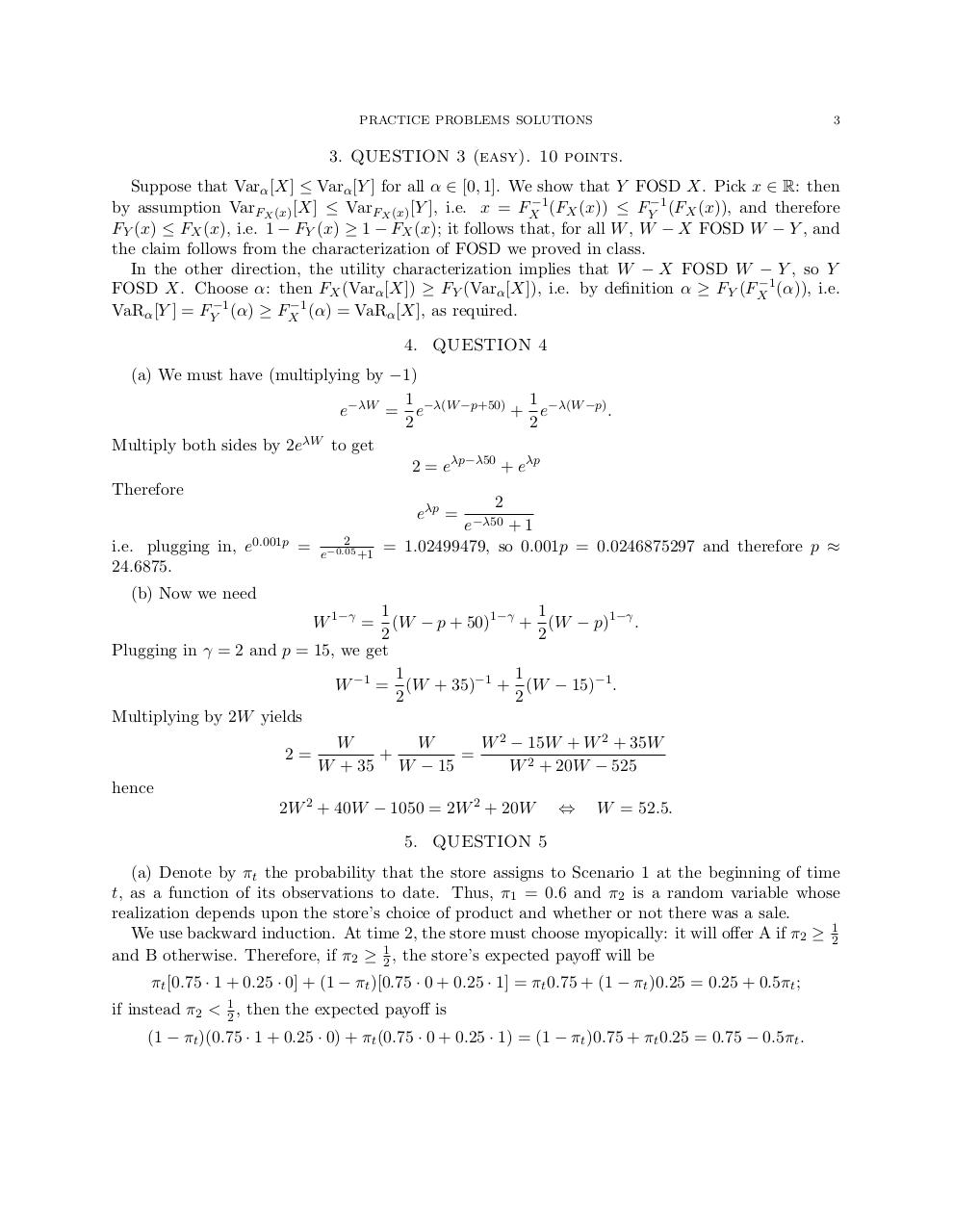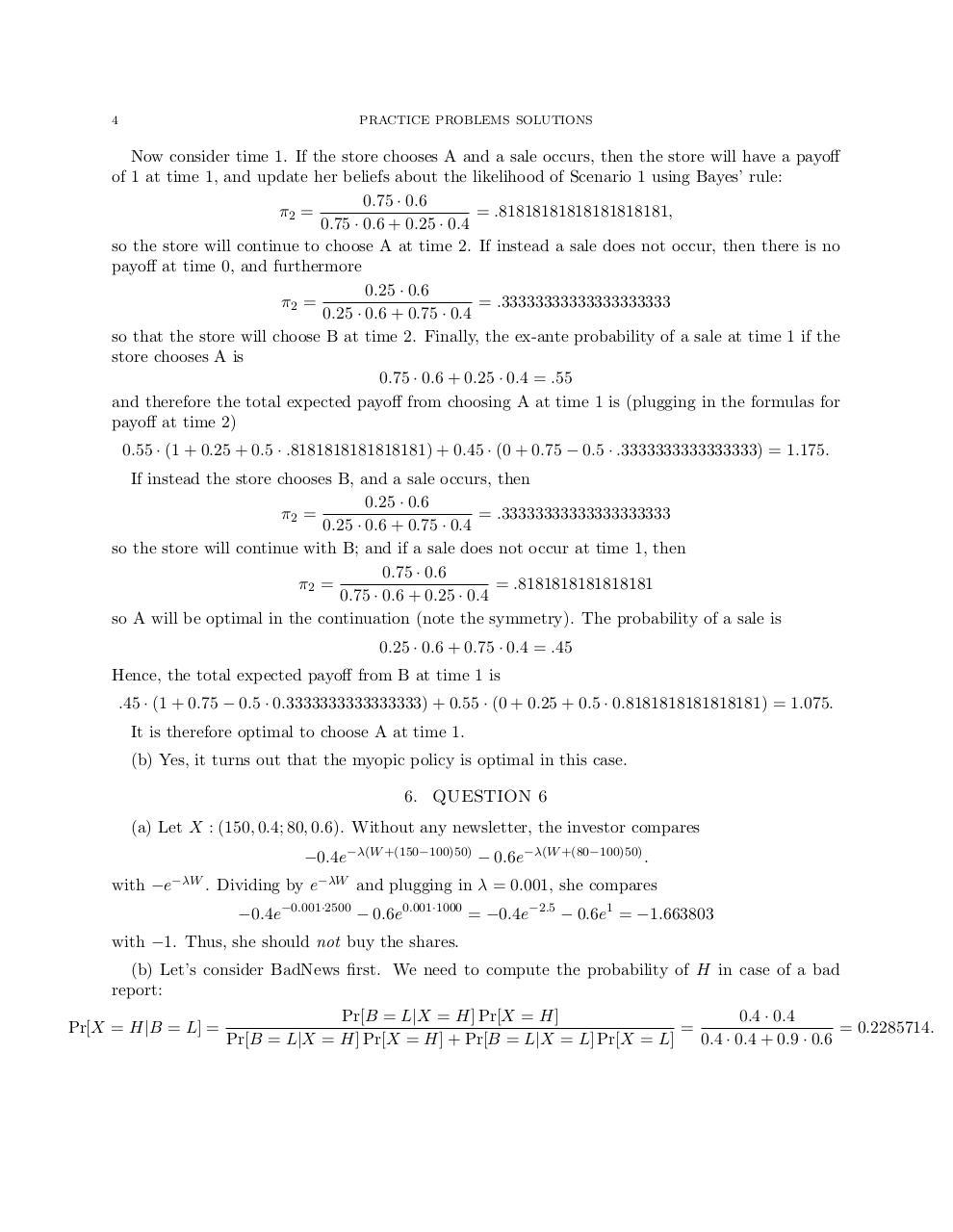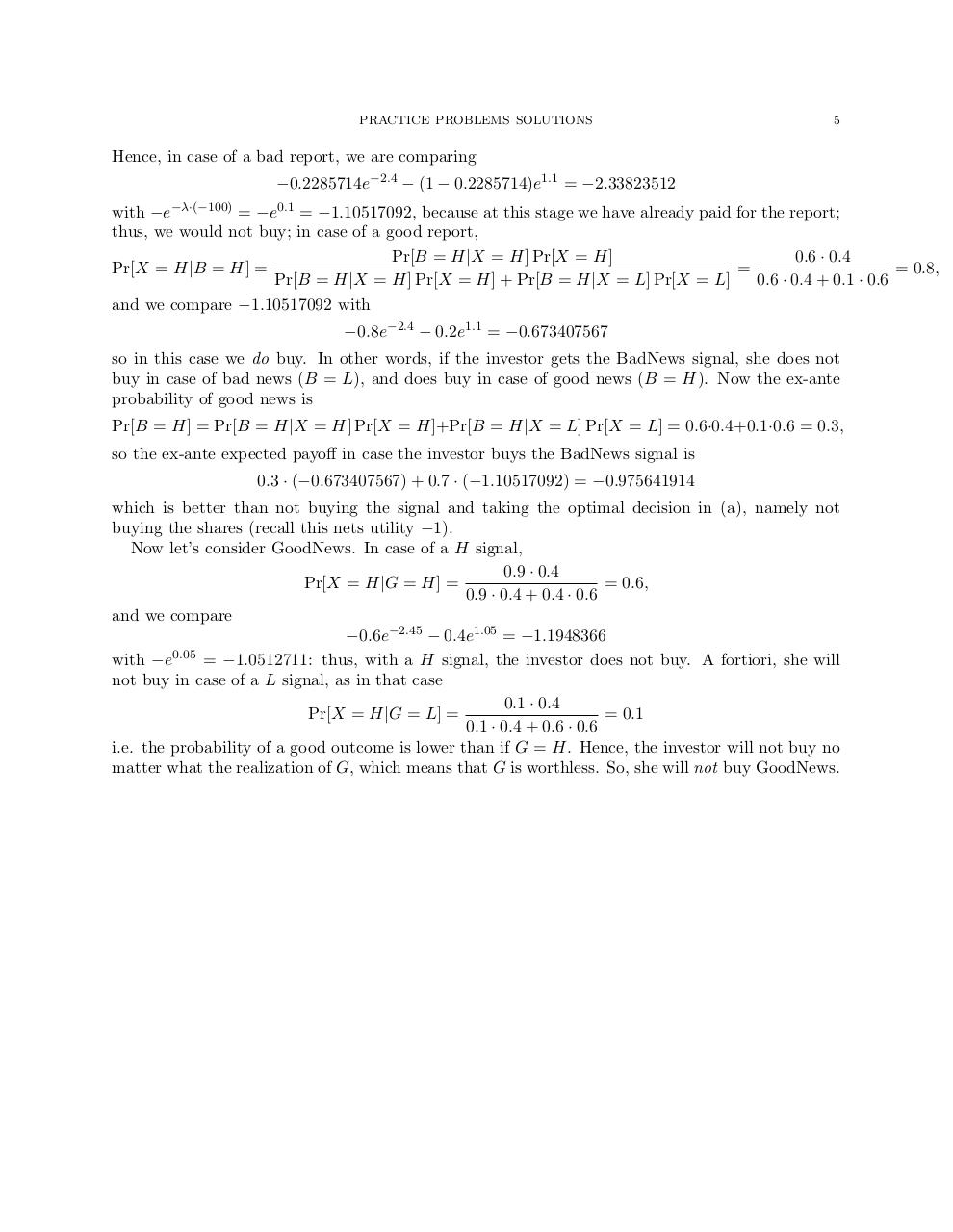#### HTML Code

Copy the following HTML code to share your document on a Website or Blog

#### QR Code### Related keywords